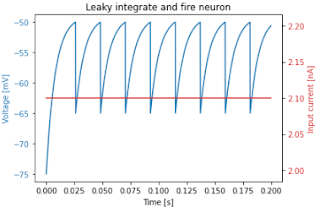Wednesday, 8 December 2021

Time to retire my Rapsberry Pi Tensorflow Docker project?

Six years ago I did some experiments using TensorFlow on the Raspberry Pi.

It takes hours to compile TensorFlow on the Pi, and when I started the Pi platform wasn't officially supported. Sam Abrahams found his way thorough the rather scary compilation process, and I used his wheel to build a Docker image for the Pi that contained TensorFlow and Jupyter. That made it easy for users to experiment by installing Docker and then running the image.

I was a bit anxious, as that was my first docker project, but it proved very popular.

Things have change a lot since then. For a while, the TensorFlow team offered official support for the Raspberry Pi, though that has now stopped. You can still download a wheel but it's very out-of-date.

I recently discovered Leigh Johnson's post on how to install full TensorFlow on the Pi. It's slightly out-of-date but the instructions on how to compile it yourself probably still work.

Most Pi-based AI projects now use TensorFlow Lite with or without the Coral USB accelerator, and I'm wondering what to do about my Docker-based Pi project.

Should I

1. Announce that work has stopped, and explain why, or
2. Try to update the project with a bulls-eye docker image containing TensorFlow 2 and Jupyter?
If you don't feel like commenting below. I'm running a poll on Twitter.

Thursday, 2 December 2021

Timings and Code for Spiking Neural Networks with JAX

I've been encouraged to flesh out my earlier posts about JAX to support 27DaysOfJAX.

I've written simulations of a Leaky Integrate and Fire Neuron in *Plowman's* (pure) Python, Python + numpy, and Python + JAX.

Here's a plot of a 2000-step simulation for a single neuron:Plot for a single neuron

The speedups using Python, Jax and the JAX jit compiler are dramatic.

Pure Python can simulate a single step for a single neuron in roughly 0.25 µs. so 1,000,000 neurons would take about 0.25 seconds.

numpy can simulate a single step for 1,000,000 neurons in 13.7 ms.

Python, JAX + JAX's jit compilation can simulate a single step for 1,000,000 neurons in 75 µs.

Here's the core code for each version.

# Pure Python
def step(v, tr, injected_current):
spiking = False
if tr > 0:
next_v = reset_voltage
tr = tr - 1
elif v > threshold:
next_v = reset_voltage
tr = int(refactory_period / dt)
spiking = True
else:
dv = ((resting_potential - v) + (injected_current * membrane_resistance)) * (dt / tau_m)
next_v = v + dv
return next_v, tr, spiking

# numpy
import numpy as np

def initial_state(count):
potentials = np.full(count, initial_potential)
ts = np.zeros(count)
injected_currents = na * (np.array(range(count)) + 1)
return injected_currents, potentials, ts

def step(v, tr, injected_current):
rv = np.full_like(v, reset_voltage)
dv = ((resting_potential - v) + (injected_current * membrane_resistance)) * (dt / tau_m)
spikes = v > threshold
next_v = np.where(spikes, rv, v + dv)
refactory = tr > 0
next_v = np.where(refactory, rv, next_v)
next_tr = np.where(refactory, tr - 1, tr)
R_DUR = int(refactory_period / dt)
next_tr = np.where(spikes, R_DUR, next_tr)
return next_v, next_tr, spikes

# JAX (the only difference from numpy is the import)
import jax.numpy as np

def initial_state(count):
potentials = np.full(count, initial_potential)
ts = np.zeros(count)
injected_currents = na * (np.array(range(count)) + 1)
return injected_currents, potentials, ts

def step(v, tr, injected_current):
rv = np.full_like(v, reset_voltage)
dv = ((resting_potential - v) + (injected_current * membrane_resistance)) * (dt / tau_m)
spikes = v > threshold
next_v = np.where(spikes, rv, v + dv)
refactory = tr > 0
next_v = np.where(refactory, rv, next_v)
next_tr = np.where(refactory, tr - 1, tr)
next_tr = np.where(spikes, R_DUR, next_tr)
return next_v, next_tr, spikes

# JAX jitting
from jax import jit

jstep = jit(step)

Jupyter Notebooks containing the full code can be found at https://github.com/romilly/spiking-jax

All the timings were run on an Intel® Core™ i5-10400F CPU @ 2.90GHz with 15.6 GiB of RAM and a NVIDIA GeForce RTX 3060/PCIe/SSE2 running Linux Mint 20.2, JAX 0.2.25 and jaxlib 0.1.73 with CUDA 11 and CUDANN 8.2.

I have successfully run the code on a Jetson Nano 4 Gb.

I've added the Nano timings to the GitHub repository.

Related posts: